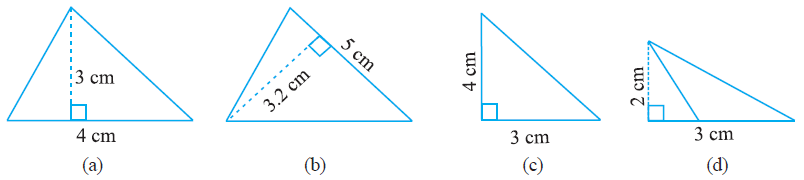# Ex.11.2 Q2 Perimeter and Area - NCERT Maths Class 7

Go back to  'Ex.11.2'

## Question

Find the area of each of the following triangles:Video Solution
Perimeter And Area
Ex 11.2 | Question 2

## Text Solution

What is known?

Base and height of triangles.

What is the unknown?

The area of the triangles.

How can you use the known information to arrive at the solution?

Base and height of a triangle is already given. Area of triangle is equal to half of product of base and height.

Steps:

(a) Given,

Base $$= 3 \,\rm cm$$

Height $$= 4 \,\rm cm$$

We know that

\begin{align}&\text{Area of triangle} \\ &= \frac{1}{2} \times {\rm{Base}} \times {\rm{Height}} \end{align}

\begin{align}&= \frac{1}{2} \times {\rm{3}} \times {\rm{4}}\\&= {\rm{6}}\;{\rm{c}}{{\rm{m}}^2}\end{align}

(b) Given

Base $$= 5 \,\rm cm$$

Height $$= 3.2 \,\rm cm$$

\begin{align}&\text{Area of triangle} \\ &= \frac{1}{2} \times {\rm{Base}} \times {\rm{Height}} \end{align}

\begin{align} &= \frac{1}{2} \times {\rm{5}} \times {\rm{3}}{\rm{.2}}\\&= {\rm{8}}\;{\rm{c}}{{\rm{m}}^2}\end{align}

(c) Given

Base $$= 3 \,\rm cm$$

Height $$= 4 \,\rm cm$$

\begin{align}&\text{Area of triangle} \\ &= \frac{1}{2} \times {\rm{Base}} \times {\rm{Height}} \end{align}

\begin{align}&= \frac{1}{2} \times {\rm{3}} \times {\rm{4}}\\& = {\rm{6}}\;{\rm{c}}{{\rm{m}}^2}\end{align}

(d) Given

Base $$= 3 \,\rm cm$$

Height $$= 2 \,\rm cm$$

\begin{align}&\text{Area of triangle} \\ &= \frac{1}{2} \times {\rm{Base}} \times {\rm{Height}} \end{align}

\begin{align}& = \frac{1}{2} \times {\rm{3}} \times {\rm{2}}\\&= {\rm{3}}\;{\rm{c}}{{\rm{m}}^2}\end{align}

Learn from the best math teachers and top your exams

• Live one on one classroom and doubt clearing
• Practice worksheets in and after class for conceptual clarity
• Personalized curriculum to keep up with school